## Info

Publication number
WO2010134381A1
WO2010134381A1 PCT/JP2010/054839 JP2010054839W WO2010134381A1 WO 2010134381 A1 WO2010134381 A1 WO 2010134381A1 JP 2010054839 W JP2010054839 W JP 2010054839W WO 2010134381 A1 WO2010134381 A1 WO 2010134381A1
Authority
WO
WIPO (PCT)
Prior art keywords
sweep
signal
calculated
beat frequency
Prior art date
Application number
PCT/JP2010/054839
Other languages
French (fr)
Japanese (ja)
Inventor

Original Assignee

Priority date (The priority date is an assumption and is not a legal conclusion. Google has not performed a legal analysis and makes no representation as to the accuracy of the date listed.)
Filing date
Publication date
Priority to JP2009-121902 priority Critical
Priority to JP2009121902A priority patent/JP5468304B2/en
Application filed by 株式会社 東芝 filed Critical 株式会社 東芝
Publication of WO2010134381A1 publication Critical patent/WO2010134381A1/en

• 230000035559 beat frequency Effects 0 abstract claims description 111
• 230000000875 corresponding Effects 0 abstract claims description 23
• 230000000051 modifying Effects 0 abstract claims description 14
• 230000004044 response Effects 0 abstract claims description 10
• 238000000034 methods Methods 0 claims description 34
• 239000000284 extracts Substances 0 claims description 7
• 230000001965 increased Effects 0 claims description 5
• 230000002829 reduced Effects 0 claims description 4
• 230000001276 controlling effects Effects 0 claims 1
• 230000000694 effects Effects 0 description 18
• 239000011159 matrix materials Substances 0 description 11
• 238000000605 extraction Methods 0 description 8
• 238000009499 grossing Methods 0 description 8
• 238000004364 calculation methods Methods 0 description 7
• 239000011651 chromium Substances 0 description 6
• 239000000562 conjugates Substances 0 description 6
• 230000001702 transmitter Effects 0 description 6
• 230000001131 transforming Effects 0 description 5
• 238000005516 engineering processes Methods 0 description 4
• 238000009740 moulding (composite fabrication) Methods 0 description 4
• 238000001228 spectrum Methods 0 description 4
• 238000007792 addition Methods 0 description 3
• 238000006243 chemical reaction Methods 0 description 3
• 238000004891 communication Methods 0 description 3
• 238000005225 electronics Methods 0 description 3
• 238000009826 distribution Methods 0 description 2
• 238000000926 separation method Methods 0 description 2
• 230000015572 biosynthetic process Effects 0 description 1
• 230000002708 enhancing Effects 0 description 1
• 238000005755 formation Methods 0 description 1
• 230000014509 gene expression Effects 0 description 1
• 230000001976 improved Effects 0 description 1
• 230000001603 reducing Effects 0 description 1
• 238000005070 sampling Methods 0 description 1
• 230000017105 transposition Effects 0 description 1

## Images

•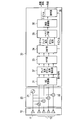•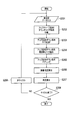•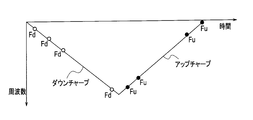•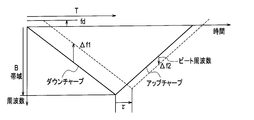•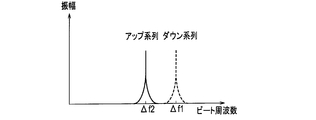•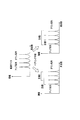•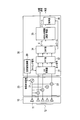•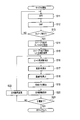•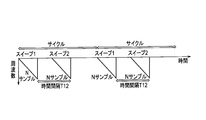•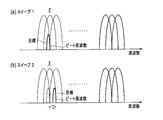•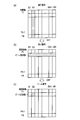•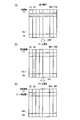•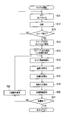•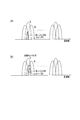•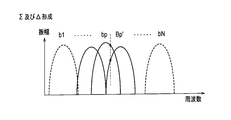•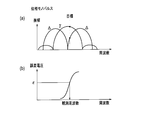•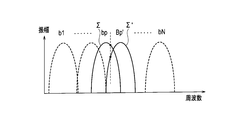•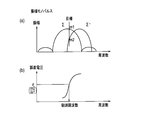•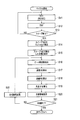•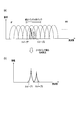•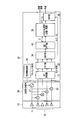•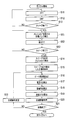•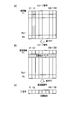•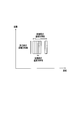•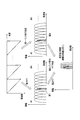•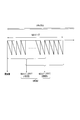•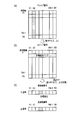•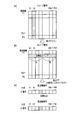•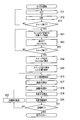•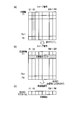•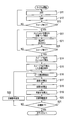•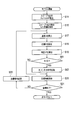•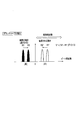•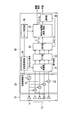•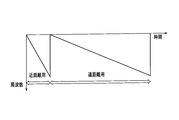•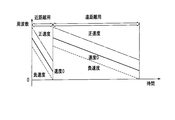•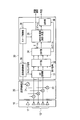•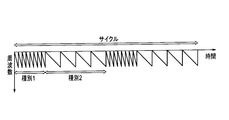•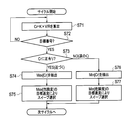•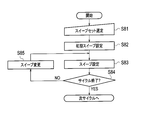•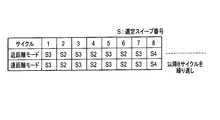•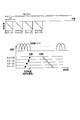••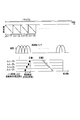•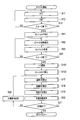•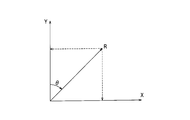•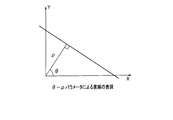•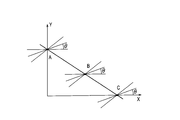••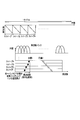•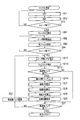## Classifications

• GPHYSICS
• G01MEASURING; TESTING
• G01S13/00Systems using the reflection or reradiation of radio waves, e.g. radar systems; Analogous systems using reflection or reradiation of waves whose nature or wavelength is irrelevant or unspecified
• G01S13/02Systems using reflection of radio waves, e.g. primary radar systems; Analogous systems
• G01S13/50Systems of measurement based on relative movement of target
• G01S13/58Velocity or trajectory determination systems; Sense-of-movement determination systems
• G01S13/583Velocity or trajectory determination systems; Sense-of-movement determination systems using transmission of continuous unmodulated waves, amplitude-, frequency-, or phase-modulated waves and based upon the Doppler effect resulting from movement of targets
• G01S13/584Velocity or trajectory determination systems; Sense-of-movement determination systems using transmission of continuous unmodulated waves, amplitude-, frequency-, or phase-modulated waves and based upon the Doppler effect resulting from movement of targets adapted for simultaneous range and velocity measurements
• GPHYSICS
• G01MEASURING; TESTING
• G01S13/00Systems using the reflection or reradiation of radio waves, e.g. radar systems; Analogous systems using reflection or reradiation of waves whose nature or wavelength is irrelevant or unspecified
• G01S13/02Systems using reflection of radio waves, e.g. primary radar systems; Analogous systems
• G01S13/06Systems determining position data of a target
• G01S13/42Simultaneous measurement of distance and other co-ordinates
• G01S13/44Monopulse radar, i.e. simultaneous lobing
• GPHYSICS
• G01MEASURING; TESTING
• G01S13/00Systems using the reflection or reradiation of radio waves, e.g. radar systems; Analogous systems using reflection or reradiation of waves whose nature or wavelength is irrelevant or unspecified
• G01S13/02Systems using reflection of radio waves, e.g. primary radar systems; Analogous systems
• G01S2013/0236Special technical features
• G01S2013/0245Radar with phased array antenna
• G01S2013/0263Passive array antenna
• GPHYSICS
• G01MEASURING; TESTING
• G01S3/00Direction-finders for determining the direction from which infrasonic, sonic, ultrasonic, or electromagnetic waves, or particle emission, not having a directional significance, are being received
• G01S3/02Direction-finders for determining the direction from which infrasonic, sonic, ultrasonic, or electromagnetic waves, or particle emission, not having a directional significance, are being received using radio waves
• G01S3/74Multi-channel systems specially adapted for direction-finding, i.e. having a single antenna system capable of giving simultaneous indications of the directions of different signals

## Abstract

Provided is a device equipped with a transceiver (20) whereby sweep signals subjected to FMCW modulation is transmitted at least twice, an FFT unit (32) whereby at least two rounds of sweep signals received in response to transmission from the transceiver are subjected to fast Fourier transforms, and an MRAV processing unit (35a) whereby beat frequencies corresponding to each of the at least two rounds of sweeps by the transceiver are calculated on the basis of at least two rounds of sweep signals obtained by performing Fourier transforms in the FFT unit, whereby velocities are calculated on the basis of beat frequency differences and time differences that are calculated, and whereby distances are calculated on the basis of velocities and beat frequencies that are calculated, thereby making calculations of distances and velocities of a plurality of targets.

## Description

The present invention relates to a radar apparatus that observes the distance and speed of a vehicle by an FMCW (Frequency-Modulated-Continuous Wave) method.

The FMCW method is known as a simple radar method for observing a vehicle traveling on a road with a radar device (for example, see Non-Patent Document 1). In the case of a radar apparatus employing this FMCW method, the distance and speed are unknown. For this reason, as a transmission / reception waveform, two parameters are generally calculated simultaneously by combining up-chirp and down-chirp.

However, on the beat frequency axis of signals transmitted and received between up-chirp and down-chirp, the frequency is different even if the target is the same. For this reason, when only a single target exists, a response can be taken. However, when there are multiple targets, there is a problem that it is difficult to pair up-chirp and down-chirp for each target. Further, since it is necessary to transmit and receive up-chirp and down-chirp, there is a problem that the cycle time becomes long.

Also, when there is a restriction on the frequency band related to the distance resolution, or when there is a restriction on the antenna aperture length related to the angular resolution, there is a problem that the separation performance of the dense target is restricted.

In addition to these problems, if the integral number N is small, in the case of the same PRF (Pulse Repetition Frequency), each beat frequency axis after FFT (Fast Fourier Transform) of the signal The frequency bank width (PRF / N) becomes large and the frequency resolution deteriorates, and there is a problem that the accuracy of distance and speed calculated based on the frequency deteriorates.

In addition, when a complex signal is extracted by performing a complex Fourier transform on a real signal and only a positive (or negative) frequency is extracted, it is correct when the correct sign of the target beat frequency is negative (or positive). There is a problem that distance, speed and angle cannot be calculated.

Further, since the beat frequency is close to a direct current (frequency is 0) component at a short distance, the beat frequency is separated from the direct current even at a short distance by increasing the frequency gradient (frequency band B / sweep time T). Need to be frequency. In this case, when the frequency band B and the sample frequency PRF are limited, there is a problem that the integral number N cannot be increased. In particular, when observing a long-distance target, if the integral number decreases, the SN ratio (signal / noise ratio) decreases, so that the detection performance and accuracy deteriorate.

FIG. 1 is a system diagram showing the configuration of a conventional radar apparatus, and FIG. 2 is a flowchart showing the operation of the radar apparatus. The radar apparatus includes an antenna 10, a transceiver 20 and a signal processor 30.

The signal swept by the transmitter 21 inside the transceiver 20 is transmitted from the antenna transmission element 11. On the other hand, the signals received by the plurality of antenna receiving elements 12 are frequency-converted by the plurality of mixers 22 and sent to the signal processor 30. In the signal processor 30, the beat frequency signal from the transmitter / receiver 20 is converted into a digital signal by the AD converter 31, and sent to the up sequence down sequence extraction unit 37 as an element signal (step S201). FIGS. 3 and 4 show transmission / reception up-chirp and down-chirp sweep signals.

The up-sequence down-sequence extraction unit 37 separates the up-chirp signal and the down-chirp signal from the element signal (digital signal) transmitted from the AD converter 31 and sends it to the FFT unit 33 (step S202). The FFT unit 33 performs fast Fourier transform on the up-chirp signal and the down-chirp signal sent from the up-sequence down-sequence extraction unit 37 to convert them into a signal on the frequency axis, and DBF (Digital Beam Forming) Send to part 34.

The DBF unit 34 forms a Σ beam (up sequence and down sequence) and a Δ beam using the frequency-axis signal sent from the FFT unit 33 (step S203). The Σ beam formed by the DBF unit 34 is sent to the pairing unit 38, and the Δ beam is sent to the angle measuring unit 36. The pairing unit 38 extracts a frequency having an extreme amplitude as shown in FIG. 5 based on the result of FFT of the up-sequence and down-sequence signals of the Σ beam (step S204). This relational expression is shown in the following expression.

here,
Δf1; Observation frequency of down-chirp signal Δf2; Observation frequency of up-chirp signal fd; Doppler frequency fr;

When the expression (3) is expanded with the target distance R and the target speed V and the expression (2) is substituted, the following expression is obtained.

here,
B: Frequency band R; Target distance T; Sweep time c; Light speed V; Target speed λ; Wavelength When the above processing is completed, up-sequence / down-sequence pairing is performed (step S205). That is, since the peak frequencies of the down chirp sequence and the up chirp sequence are different, processing for associating the frequency pair is performed. Next, the target distance and speed are calculated (step S206), and the angle is calculated (step S207).

Thereafter, it is checked whether or not the cycle is completed (step S208). If it is determined in step S208 that the cycle has not ended, processing for moving to the next cycle is performed (step S209). Thereafter, the process returns to step S201, and the above-described process is repeated. On the other hand, if the cycle ends in step S208, the processing of the radar device ends.

The target distance R and speed V can be calculated by the above processing. As described above, since the peak frequencies of the down-chirp sequence and the up-chirp sequence are different, it is necessary to correspond the frequency pair. Pairing is relatively easy in the case of a single goal or a minority goal. However, when the target number and the reflection point of the background increase, as shown in FIG. 6, there is a problem that the number of peak values on the frequency axis increases and pairing becomes difficult.

Supervised by Takashi Yoshida, "Revised Radar Technology", IEICE, pp.274-275 (1996)

As described above, the conventional radar apparatus has the following problems.

(1) When combining up-chirp and down-chirp signals as transmission / reception waveforms, if there are multiple targets, pairing is difficult. In addition, since it is necessary to transmit and receive up-chirp and down-chirp signals, the cycle time becomes long.

(2) When the distance resolution or the angular resolution is limited, the separation performance of the dense target is limited.

(3) When the integral number N is small, in the case of the same PRF, each frequency bank width of the beat frequency axis after the fast Fourier transform becomes large and the frequency resolution deteriorates, and the distance and speed calculated based on the frequency The accuracy of is degraded.

(4) When a complex signal is extracted by performing a complex Fourier transform on a real signal to extract only a positive (or negative) frequency, it is correct if the correct sign of the target beat frequency is negative (or positive). Cannot calculate distance, speed and angle.

(5) Since the beat frequency is close to a direct current (frequency is 0) component at a short distance, it is necessary to increase the frequency gradient. In this case, if the frequency band B and the sample frequency PRF are limited, the integration number N cannot be increased.

An object of the present invention is to provide a radar apparatus that can observe a target with high detection performance and high accuracy even when a plurality of targets exist in a wide range from a short distance to a long distance.

In order to solve the above-mentioned problems, the first invention is a transmitter / receiver for transmitting an FMCW-modulated sweep signal M times, and a fast Fourier transform for M times of the sweep signal received in response to transmission from the transmitter / receiver. When the local maximum value of each sweep signal is calculated from the M sweep signal obtained by performing the Fourier transform in the FFT unit and the FFT unit, the beat frequency is calculated by the M sweep phase monopulse, amplitude monopulse or MUSIC. The result F (sweep number, target number) is used to perform amplitude integration in the sweep direction for each beat frequency on the beat frequency-sweep axis, and for each frequency bank that exceeds the predetermined threshold, the sweep that exceeds the predetermined threshold Calculate the least square line between the relative distance of the number and the sweep time, and set the target speed by the gradient of the least square line. Out, and a MRAV processing unit for calculating the distance of the target.

According to a second aspect of the present invention, there is provided a transceiver for transmitting an FMCW modulated sweep signal at least twice, an FFT unit for fast Fourier transforming at least two sweep signals received in response to transmission from the transceiver, and an FFT. The beat frequency corresponding to each of at least two sweeps by the transmitter / receiver is calculated based on at least two sweep signals obtained by performing Fourier transform in the unit, and the speed is calculated based on the calculated beat frequency difference and time difference. And an MRAV processing unit that calculates distances and speeds of a plurality of targets by calculating a distance based on the calculated speed and beat frequency.

A third invention includes a transceiver that transmits an FMCW-modulated sweep signal M times, an FFT unit that fast Fourier transforms M sweep signals received in response to transmission from the transceiver, and an FFT unit. When calculating the maximum value of each sweep signal from the M sweep signals obtained by Fourier transform, the result F (sweep number, target) of the beat frequency calculated by the phase monopulse, amplitude monopulse or MUSIC of the M sweep And an MRAV processing unit that calculates a distance after calculating the speed based on the smoothed result.

A fourth invention includes a transceiver that transmits an FMCW-modulated sweep signal M times, an FFT unit that fast Fourier transforms M sweep signals received in response to transmission from the transceiver, and an FFT unit. When calculating the maximum value of each sweep signal from the M sweep signals obtained by Fourier transform, the result F (sweep number, target) of the beat frequency calculated by the phase monopulse, amplitude monopulse or MUSIC of the M sweep MRAV processing unit that calculates the maximum value by Hough transform on the beat frequency-sweep axis using the number), calculates the speed corresponding to the calculated maximum value from the beat frequency difference and the sweep time, and then calculates the distance With.

According to the first invention, by integrating the amplitude in the sweep direction for each beat frequency on the beat frequency-sweep axis, an integration effect between a plurality of sweeps is obtained to improve the signal detection performance. In addition, the distance is calculated after calculating the inclination of the straight line extracted by fitting with the least square line and calculating the velocity. Thereby, even when there is an error in the relative distance difference, the influence of the error can be reduced, and the accuracy of speed measurement and distance measurement can be improved.

According to the second invention, it is not necessary to perform pairing in the case of a plurality of targets unlike the conventional radar apparatus, and radar observation with a short cycle time can be realized.

According to the third invention, since the speed and distance are calculated by smoothing between sweeps, even if there is an error in the relative distance difference, the influence of the error is reduced and the accuracy of speed measurement and distance measurement is improved. be able to.

According to the fourth aspect of the invention, by performing the Hough transform on the beat frequency-sweep axis, an integration effect between a plurality of sweeps is obtained to improve the signal detection performance. Also, the distance is calculated after calculating the speed by calculating the slope of the straight line extracted by the Hough transform. Thereby, even when there is an error in the relative distance difference, the influence of the error can be reduced and the accuracy of speed measurement and distance measurement can be improved.

Hereinafter, embodiments of the present invention will be described in detail with reference to the drawings. The radar apparatus according to the present invention employs a simple system in which pairing is performed only between the same frequency bank or adjacent banks using a continuous FMCW signal that is easy to mount.

The radar apparatus according to the first embodiment of the present invention employs an MRAV (Measurement Range After Measurement Velocity) method of calculating a distance after calculating a velocity based on a beat frequency. FIG. 7 is a system diagram showing the configuration of the radar apparatus according to Embodiment 1 of the present invention. This radar apparatus includes an antenna 10, a transceiver 20, and a signal processor 30a.

The antenna 10 includes an antenna transmitting element 11 and a plurality of antenna receiving elements 12. The antenna transmission element 11 converts a transmission signal sent as an electrical signal from the transceiver 20 into a radio wave and sends it out. The plurality of antenna receiving elements 12 receive external radio waves, convert them into electrical signals, and send them to the transceiver 20 as received signals.

The transceiver 20 includes a transmitter 21 and a plurality of mixers 22, and the plurality of mixers 22 are provided corresponding to the plurality of antenna receiving elements 12, respectively. The transmitter 21 generates a transmission signal in accordance with the transmission control signal sent from the signal processor 30 and sends it to the antenna transmission element 11 and the plurality of mixers 22. The plurality of mixers 22 frequency-convert the received signals received from the plurality of antenna receiving elements 12 in accordance with the signals from the transmitter 21 and send the signals to the signal processor 30.

The signal processor 30a includes an AD converter 31, an FFT unit 32, a DBF unit 34, an MRAV processing unit 35a, an angle measuring unit 36, and a transmission / reception control unit 39.

The AD converter 31 converts the analog signal sent from the transmitter / receiver 20 into a digital signal according to the timing signal sent from the transmission / reception control unit 39, and sends it to the FFT unit 32 as an element signal.

The FFT unit 32 converts the element signal sent from the AD converter 31 into a signal on the frequency axis by fast Fourier transform, and sends it to the DBF unit 34.

The DBF unit 34 uses the signals on the frequency axis sent from the FFT unit 33 to form a Σ beam and a Δ beam. The Σ beam formed by the DBF unit 34 is sent to the MRAV processing unit 35a, and the Δ beam is sent to the angle measuring unit 36.

The MRAV processing unit 35a performs ranging and speed measurement based on the Σ beam from the DBF unit 34. The distance and speed obtained by the distance measurement and speed measurement in the MRAV processing unit 35a are output to the outside.

The angle measuring unit 36 performs angle measurement based on the Δ beam sent from the DBF unit 34. The angle obtained by the angle measurement by the angle measuring unit 36 is output to the outside.

The transmission / reception control unit 39 generates a transmission control signal for starting transmission and sends the transmission control signal to the transmitter 21 of the transmitter / receiver 20, and generates a timing signal that defines the timing for capturing the signal from the transmitter / receiver 20 to perform AD conversion. Send to vessel 31.

Next, the operation of the radar apparatus according to the first embodiment of the present invention configured as described above will be described with reference to the flowchart shown in FIG. 8 with a focus on measurement processing for ranging / velocity measurement and angle measurement. Is done.

In the measurement process, when a cycle is started, first, a sweep 1 that is a frequency-changed sweep signal, that is, an FM-modulated sweep signal, is transmitted from the antenna transmission element 11 and transmitted as shown in FIG. The received signal is received by the antenna receiving element 12. The received signal is frequency-converted by the transmitter / receiver 20 and sent to the AD converter 31 of the signal processor 30a. The AD converter 31 converts the analog signal sent from the transceiver 20 into a digital signal. As a result, as shown in FIG. 11A, signals of N samples corresponding to each of the time axes T1 to TN are obtained for the elements having the element numbers E1 to EM of the antenna receiving element 12. The signal obtained by the AD converter 31 is sent to the FFT unit 32 as an element signal.

In this state, fast Fourier transform (FFT) is performed (step S11). That is, the FFT unit 32 performs a fast Fourier transform on the element signal sent from the AD converter 31. As a result, as shown in FIG. 11B, the beat frequency on the frequency axis for N samples corresponding to each of the frequency axes F1 to FN for the elements to which the element numbers E1 to EM of the antenna receiving element 12 are attached. A signal is obtained. The beat frequency signal obtained by the FFT unit 32 is sent to the DBF unit 34.

Next, DBF processing is performed (step S12). That is, the DBF unit 34 forms a Σ beam and a Δ beam in the angle direction using the signal on the frequency axis sent from the FFT unit 33. Thereby, as shown in FIG.11 (c), the beam which has a peak in a specific beam number (for example, B2) is formed. The Σ beam formed by the DBF unit 34 is sent to the MRAV processing unit 35, and the Δ beam is sent to the angle measuring unit 36.

Next, it is determined whether or not the sweep is finished (step S13). That is, it is checked whether or not the processing for both sweep 1 and sweep 2 has been completed. If the sweep has not ended in step S13, the process returns to step S11, and the above-described process is repeated for sweep 2 that is the next FM-modulated sweep signal.

On the other hand, when the sweep is completed in step S13, threshold detection of sweep 1 and sweep 2 is performed (step S14). That is, the DBF unit 34 detects the threshold level of the Σ beam obtained by the sweep 1 and the sweep 2. Next, the target detected in step S14 is stored (step S15). That is, the DBF unit 34 detects and stores the target from the threshold level detected in step S14.

Next, the beat frequency is extracted (step S16). That is, as shown in FIG. 10, the MRAV processing unit 35a extracts the beat frequency fp having a peak signal and the bank signal based on the FFT and DBF results of the sweep 1 and the sweep 2.

Next, the speed V is calculated (step S17). That is, MRAV processing unit 35a calculates relative distances R1 and R2 using beat frequencies fp of sweep 1 and sweep 2, and calculates speed V = (R2-R1) / T12.

Next, the distance R is calculated (step S18).

here,

R; distance T; sweep time B; frequency band fp; beat frequency c; speed of light Based on the beat frequency fp and velocity V, the distance R and velocity V are calculated by simultaneous equations.

v: speed R1, R2: distance between sweeps 1 and 2 T12: time interval between sweeps 1 and 2 fp: beat frequency λ: wavelength B: band T: sweep time Next, an angle Θ is calculated (step S19). That is, the angle measuring unit 36 performs angle measurement based on the Δ beam sent from the DBF unit 34 and outputs the angle obtained by this angle measurement to the outside.

Next, the target information is stored (step S20). That is, the target speed V, distance R, and angle Θ calculated in step S17 are stored. Next, it is checked whether or not the goal is finished (step S21). That is, it is checked whether or not the processing for all the targets has been completed. In step S21, when the target has not ended, the target number is changed to the next number, and the process returns to step S16 and the above-described processing is repeatedly executed. On the other hand, if the target is finished in step S21, the measurement process is finished.

As described above, according to the radar apparatus according to the first embodiment of the present invention, in order to transmit / receive an up-chirp or down-chirp sequence signal, pairing in the case of multiple beat targets having the same beat frequency is performed. There is no need to do. Also, radar observation with a short cycle time can be realized.

The radar apparatus according to the first embodiment described above performs digital beam forming (DBF) after performing fast Fourier transform (FFT) to obtain the beat frequency. However, as shown in FIG. 12, digital beam forming (DBF) is performed. Then, the beat frequency can be obtained by fast Fourier transform (FFT).

The radar apparatus according to the second embodiment of the present invention employs a method in which a phase monopulse is combined with the MRAV method according to the first embodiment described above. The configuration of the radar apparatus according to the second embodiment is the same as the configuration of the radar apparatus according to the first embodiment illustrated in FIG.

FIG. 13 is a flowchart showing the operation of the radar apparatus according to the second embodiment of the present invention, centering on measurement processing for performing distance measurement / speed measurement and angle measurement. In addition, the same code | symbol as the code | symbol used in FIG. 8 is attached | subjected to the step which performs the process which is the same as the measurement process which concerns on Example 1 shown by the flowchart of FIG. 8, or an equivalent process. In the following, portions different from the first embodiment will be mainly described.

Especially, when the PRF is the same and the number of sample points is small, the frequency bank interval becomes large and the frequency accuracy deteriorates. Therefore, as a countermeasure, the radar apparatus according to the second embodiment observes the frequency in the bank with high accuracy using the phase monopulse used on the angle axis as the frequency axis as shown in FIGS. The phase monopulse (sometimes referred to as phase comparison monopulse) is described in “Supervised by Takashi Yoshida,“ Revised Radar Technology ”, IEICE, pp.274-275 (1996)”.

In monopulse distance measurement / speed measurement, as shown in FIG. 16, an error voltage εp of the following equation is calculated using Σ (f) and Δ (f) of the extracted target frequency. The phase monopulse processing is performed by the FFT unit 32.

here,
Σ: Addition (Received data 1 to N multiplied by weighting 1 and then FFT)
Δ: Subtraction (1 to N / 2 of received data is multiplied by -1 and N / 2 + 1 to N is multiplied by weighting 1 and then FFT)
*; Complex conjugate Re; Real part Next, the reference value ε0 of the error voltage εp calculated using the frequency characteristics of Σ and Δ stored in advance is tabulated (corresponding to ε0 and frequency f). Using the reference table, the beat frequency fp is extracted from the observed value ε (step S16). Then, the speed and distance are calculated using the extracted beat frequency fp (steps S17 and S18).

Regarding weighting, in addition to -1 or 1, weights such as tailor weights based on tailor distribution may be multiplied in order to reduce side lobes. The Taylor distribution is described in, for example, “Supervised by Takashi Yoshida,“ Revised Radar Technology ”, IEICE, pp.274-275 (1996)”.

As described above, according to the radar apparatus according to the second embodiment of the present invention, the beat frequency of each sweep signal is calculated with high accuracy based on the phase monopulse error voltage. It can be calculated with high accuracy.

The radar apparatus according to the third embodiment of the present invention uses an amplitude comparison monopulse instead of the phase monopulse of the radar apparatus according to the second embodiment. The configuration of the radar apparatus according to the third embodiment is the same as the configuration of the radar apparatus according to the first embodiment illustrated in FIG. Hereinafter, portions different from the first embodiment will be mainly described. The amplitude comparison monopulse (also called amplitude monopulse) is described in “Supervised by Takashi Yoshida,“ Revised Radar Technology ”, IEICE, pp.274-275 (1996)”.

Using the Σ (f), Σ (f−1) and Σ (f + 1) of the banks before and after the extracted target frequency, the absolute value abs (Σ (f−1)) and the absolute value abs (Σ (f + 1) ) And the larger one is set as the absolute value abs (Σu).

Then, an error voltage εa of the following equation is calculated (see FIGS. 17 and 18). The amplitude monopulse process is performed by the FFT unit 32.

here,
Σ: Addition (FFT after multiplying received data 1 to N by weighting 1)
Σu; the larger absolute value of (f−1) and Σ (f + 1), and the error voltage εp calculated using the frequency characteristics of the absolute values abs (Σ) and absolute values abs (Σu) stored in advance The reference value ε0 is tabulated (correspondence between ε0 and frequency f). The beat frequency value fp is extracted based on the observed value ε using the reference table. The speed and distance are calculated using the extracted beat frequency fp.

As described above, according to the radar apparatus according to the third embodiment of the present invention, the beat frequency of each sweep signal is calculated with high accuracy based on the amplitude monopulse error voltage. Can be calculated with high accuracy.

As for the weighting, a weight such as a tailor weight may be multiplied to reduce the side lobes in addition to −1 or 1 as in the radar apparatus according to the second embodiment described above.

The radar apparatus according to Embodiment 4 of the present invention employs the MUSIC method. The MUSIC method is described in “HARRY B.LEE,“ Resolution ”Threshold of Beams“ pace ”MUSIC“ For ”Two“ Closely ”Spaced“ Emitters ”,“ IEEE ”Trans.“ ASSP, ”Vol. 38,“ No. 9, “Sept.” (1990) .

The configuration of the radar apparatus according to the fourth embodiment is the same as that of the radar apparatus according to the first embodiment shown in FIG. FIG. 19 is a flowchart illustrating the operation of the radar apparatus according to the fourth embodiment of the present invention, centering on measurement processing for performing distance measurement / speed measurement and angle measurement. In the flowchart shown in FIG. 19, the same reference numerals as those used in FIG. 7 are given to steps for performing the same or corresponding processing as the measurement processing according to the first embodiment shown in the flowchart of FIG. 8. . In the following, portions different from the first embodiment will be mainly described.

In the radar apparatus according to the fourth embodiment, as illustrated in FIG. 20, when the FFT unit 32 performs a fast Fourier transform on the sweep signal, a bank signal of ± M is extracted around the maximum value bank of the Σ signal, The beat frequency is calculated with high accuracy by applying the beam space type MUSIC. This radar apparatus calculates the speed based on the beat frequency difference (distance difference) twice and the time difference, and further calculates the absolute distance based on the beat frequency and the speed, thereby calculating the distance and speed of Nt targets. calculate.

In addition, although the document explaining the above-mentioned MUSIC system describes the beam on the angle axis, the beam can be expanded on the frequency axis such as fast Fourier transform (FFT).

The procedure is as follows. That is, as shown in FIG. 20A, a target bank whose amplitude exceeds a predetermined threshold is extracted based on the result of the fast Fourier transform (FFT). Then, a correlation matrix Rbb is calculated from ± M banks (2M + 1) of complex signals Xm around the extracted target bank.

here,
Rbb: Correlation matrix X: Column vector having elements XM to X0 to XM (2M + 1) H: Complex conjugate transposition Next, the eigenvector Eb of Rbb of the correlation matrix is calculated. Then, an eigenvector EN for noise is extracted from the eigenvector Eb. Using the eigenvector EN and the steering vector w for searching for the frequency, the MUSIC spectrum is calculated by the following equation. As shown in FIG. 20 (b), the beat frequency fp having an extreme value in the spectrum is read.

here,
ws: steering column vector having element ws (n) on the time axis fs: search frequency PRF: repetition frequency n: 1 to N (N is the number of samples)
FFT []: Fourier transform EN: Eigenvector of correlation matrix Rbb H: Conjugate transposition Next, the calculated beat frequency fp is used to calculate velocity and distance (steps S17 and S18).

As described above, according to the radar apparatus according to Embodiment 4 of the present invention, the beat frequency of each sweep signal is calculated with high accuracy based on the FFT and MUSIC processing. Can be calculated with high accuracy.

FIG. 21 is a block diagram showing a configuration of a radar apparatus according to Embodiment 5 of the present invention. This radar apparatus is configured by adding a second FFT section 40 between the DBF section 34 and the MRAV processing section 35 in the signal processor 30a of the radar apparatus shown in FIG. The second FFT unit 40 performs a fast Fourier transform on the signal output from the DBF unit 34.

In the radar apparatus according to the fifth embodiment, as shown in FIGS. 23 to 25, a method of integrating between a plurality of sweeps is adopted in order to further improve the SN ratio and improve the frequency resolution within the restriction of the frequency band. Has been.

This radar device transmits an FMCW-modulated sweep signal N times (# 1 to #N), and extracts the local maximum value of the Nt point from the result of the fast Fourier transform of each sweep. As shown in FIG. 26, the radar apparatus performs two M sweeps by extracting a bank of local maximum values from FFT signals of # 1 to # N1 (M sweep) and # N2 to #N (M sweep) sweeps. The beat frequency of the bank signal having the maximum value among the results of each fast Fourier transform is calculated. The radar apparatus calculates the speed based on the two beat frequency differences (distance difference) and the time difference, and calculates the absolute distance based on the beat frequency and the speed, thereby calculating the distance and speed of the Nt targets. To do.

FIG. 27 shows the state of each FFT in the two M sweeps. After the first N-sample FFT is performed, a second M-sample FFT is performed. At this time, the first FFT unit 32 calculates Δ (difference between 1 to N / 2 and N / 2 + 1 to N) in addition to the sum Σ of N samples. In the second FFT unit 40, a Σ and Δ signal by two-stage FFT is obtained by calculating the sum of Σ and Δ of M samples as a result of the first FFT. Thereafter, a phase monopulse calculation may be performed to calculate a highly accurate frequency fp.

FIG. 22 is a flowchart showing the operation of the radar apparatus according to the fifth embodiment of the present invention, centering on measurement processing for performing distance measurement / speed measurement and angle measurement. In the flowchart of FIG. 22, the same reference numerals as those used in FIG. 8 are given to steps for performing the same or corresponding processes as the measurement process according to the first embodiment shown in the flowchart of FIG. 8. In the following, a description will be given centering on differences from the first embodiment.

First, N sweeps are transmitted and received, and the first FFT is executed by the FFT unit 32 for each sweep (steps S11 to S13). Next, a target bank exceeding a predetermined threshold is extracted (step S51). Next, the target bank of each sweep is subjected to a second FFT by the second FFT unit 39 (step S52). Thereafter, the peak beat frequency fp is read. Then, speed calculation (step S17) and distance calculation (step S18) are performed using the calculated beat frequency fp.

In the case of a phase monopulse, the following processing is performed. First, N sweeps are transmitted and received, and the first FFT is executed by the FFT unit 32 for each sweep (steps S11 to S13). Next, the Σ signal and the Δ signal are calculated, and a target bank in which the absolute value of the Σ signal exceeds a predetermined threshold is extracted (step S51). Next, the Σ signal and Δ signal of the target bank of each sweep are subjected to a second FFT by the second FFT unit 40 (step S52). Then, the frequency fp is calculated using the Σ signal and the Δ signal, and the distance and the speed are calculated using the calculated frequency fp.

As described above, according to the radar apparatus of the fifth embodiment of the present invention, the extracted bank signal of each sweep signal is further subjected to the second FFT between sweeps, so that the resolution is higher than that of the bank using only the first FFT. The target position and speed can be extracted.

In the radar apparatus according to Embodiment 6 of the present invention, when each local maximum value is calculated from two M sweep signals in the radar apparatus according to Embodiment 5, as shown in FIG. , Σ and Δ are calculated, and the beat frequency is calculated with high accuracy based on the monopulse error voltage.

FIG. 29 is a flowchart showing the operation of the radar apparatus according to Embodiment 6 of the present invention, centering on measurement processing for measuring distance / speed measurement and angle measurement. In the flowchart of FIG. 26, the same reference numerals as those used in FIG. 22 are assigned to steps that perform the same or corresponding processes as the measurement process according to the fifth embodiment shown in the flowchart of FIG. In the following, a description will be given centering on differences from the fifth embodiment.

First, N sweeps are transmitted and received, and FFT is executed for each sweep (steps S11 to S13). Next, a target bank whose amplitude exceeds a predetermined threshold is extracted (step S51). Next, Σ and Δ are calculated by the second FFT unit 40 using the target banks E1 to EM extracted in step S51. Then, an error voltage εp of the following equation is calculated using Σ (f) and Δ (f) of the extracted target frequency.

Σ: Addition (FFT after multiplying E1 to EM by weight 1)
Δ: Subtraction (E1 to EM / 2 multiplied by −1, EM / 2 + 1 to EM weighted by 1 and then FFT)
*; Complex conjugate Re; Real part Next, the reference value ε0 of the error voltage εp calculated using the frequency characteristics of Σ and Δ stored in advance is tabulated (corresponding to ε0 and the frequency f). Using the reference table, the frequency value fp is extracted based on the observed value ε (step S16). Then, speed calculation (step S17) and distance calculation (step S18) are performed using the extracted beat frequency fp.

As described above, according to the radar apparatus according to Embodiment 6 of the present invention, when performing the second FFT by the second FFT unit 40, the beat frequency is calculated with high accuracy by calculating the frequency using the phase monopulse. The target position and speed can be extracted with high accuracy.

As for the weighting, a weight such as a tailor weight may be multiplied to reduce the side lobes in addition to −1 or 1 as in the radar apparatus according to the second embodiment described above.

In the radar apparatus according to Embodiment 7 of the present invention, when each local maximum value is calculated from the M sweep signal in the radar apparatus according to Embodiment 6, Σ and Σu of the M sweep are calculated as shown in FIG. The beat frequency is calculated with high accuracy from the monopulse error voltage.

First, N sweeps are transmitted and received, and the first FFT is executed for each sweep (steps S11 to S13). Next, a target bank whose amplitude exceeds a predetermined threshold is extracted (step S51). Next, using the Σ (f), Σ (f−1) and Σ (f + 1) of the banks before and after the extracted target frequency, the absolute value abs (Σ (f−1)) and the absolute value abs (Σ (F + 1)) are compared, and the larger one is set as the absolute value abs (Σu).

Then, an error voltage εa of the following equation is calculated. The monopulse error voltage process is performed by the second FFT unit 40 (step S61).

here,
Σ: Addition (FFT after multiplying received data 1 to N by weighting 1)
Σu; the larger absolute value of Σ (f−1) and Σ (f + 1), and the reference value of the error voltage εp calculated using the frequency characteristics of abs (Σ) and abs (Σu) stored in advance ε0 is tabulated (corresponding to ε0 and frequency f). The beat frequency value fp is extracted based on the observed value ε using the reference table. The distance and speed are calculated using the calculated beat frequency fp.

As described above, according to the radar apparatus according to the seventh embodiment of the present invention, when performing the second FFT by the second FFT unit 40, the beat frequency is calculated with high accuracy by calculating the frequency using the amplitude monopulse. The target position and speed can be extracted with high accuracy.

As for the weighting, a weight such as a tailor weight may be multiplied by a weight other than −1 or 1 in order to reduce the side lobe, similarly to the radar apparatus according to the second embodiment described above.

As shown in FIG. 30, the radar apparatus according to Example 8 of the present invention calculates the beat frequency by FFT and MUSIC processing of M sweep when calculating each local maximum value from two M sweep signals. .

FIG. 31 is a flowchart showing the operation of the radar apparatus according to the eighth embodiment of the present invention, centering on measurement processing for measuring distance / speed measurement and angle measurement. In the flowchart of FIG. 31, the same reference numerals as those used in FIG. 22 are given to steps for performing the same or corresponding processes as the measurement process according to the fifth embodiment shown in the flowchart of FIG. 22. In the following, a description will be given centering on differences from the fifth embodiment.

First, a target bank whose amplitude exceeds a predetermined threshold is extracted based on the result of FFT in steps S11 to S13 (step S51). Next, a correlation matrix Rbb is calculated based on the complex signal Xm for M sweeps of the target bank.

here,
Rbb: Correlation matrix X: Column vector having elements X1 to XM (M) H: Complex conjugate transposition Next, an eigenvector Eb of the correlation matrix Rbb is calculated. Then, an eigenvector EN for noise in the eigenvector Eb is extracted, a MUSIC spectrum is calculated by the following equation using the eigenvector EN and a steering vector w for searching for a frequency, and the beat frequency at which the spectrum has an extreme value is calculated. fp is read. This MUSIC process is performed by the second FFT unit 39 (step S71).

here,
ws; steering column vector having element ws (n) on the time axis fs: search frequency Fs: 1 / sweep time interval n: 1 to N (N is the number of samples)
FFT []: Fourier transform EN: Eigenvector of correlation matrix Rbb H: Conjugate transposition Next, speed calculation (step S17) and distance calculation (step S18) are performed using the calculated beat frequency fp.

As described above, according to the radar apparatus according to the eighth embodiment of the present invention, when the second FFT is performed by the second FFT unit 40, the frequency is calculated by the FFT and MUSIC processing, so that the beat can be accurately performed. The frequency can be extracted, and the target position and speed can be extracted with high accuracy.

When the sweep signal is a real signal (not a complex signal), the radar apparatus according to Embodiment 9 of the present invention extracts a positive (or negative) signal from the beat frequency by performing a complex Fourier transform on the sampled signal. Thus, a complex signal is obtained. In this case, depending on the distance and speed, the target is actually observed as positive (or negative) even if the beat frequency is negative (or positive), and therefore the distance and speed may be wrong. In this case, it can be determined that the beat frequency is negative (positive) based on the following principle.

According to the MRAV method, the moving speed is observed at v ′ = − v (R2 <R1) <0 (see FIG. 33). If the beat frequency is actually negative, the observed frequency is
fp ′ = − 2v / λ + 2R · B / cT> 0
It is. Therefore,
fp ′ + 2v ′ / λ = 2R · B / cT
From the third equation (6), R ′ = positive · (fp ′ + 2v ′ / λ) = positive × 2R · B / cT <0
Therefore, the distance R ′ in the above expression is negative, and when R ′ is negative, it can be determined that the speed is increasing. If it can be determined that the speed is negative, the signs of v ′, fp ′ and R ′ are reversed, and normal values of v and R are calculated.

FIG. 32 is a flowchart showing the operation of the radar apparatus according to Embodiment 9 of the present invention, centering on measurement processing for measuring distance / speed measurement and angle measurement. In the flowchart of FIG. 32, the same reference numerals as those used in FIG. 8 are assigned to steps that perform the same or corresponding processes as the measurement process according to the first embodiment shown in the flowchart of FIG. In the following, a description will be given centering on differences from the fifth embodiment.

The negative velocity observation method by the MRAV method is performed by the following procedure. After the distance difference between the two sweeps is observed, the target velocity V is calculated based on the position change and time (step S17). Next, the distance R is calculated from the beat frequency using the target speed V calculated in step S17 (step S18). Next, the angle Θ is calculated (step S19). Next, it is checked whether or not the distance R is negative (step S61). If the distance R is negative in step S61, the sign inversion unit (not shown) inverts the signs of the distance R, the speed V, and the angle Θ (step S62). On the other hand, if the distance R is not negative in step S61, the process of step S62 is skipped.

As described above, according to the radar apparatus according to the ninth embodiment of the present invention, when a real sampling frequency is subjected to complex Fourier transform and only a positive (or negative) beat frequency is observed, a true target signal is obtained. Even when the beat frequency has a negative (or positive) beat frequency, the correct sign can be determined based on the calculated sign of the distance and converted to the correct sign of the distance, speed, and angle.

The radar apparatus according to Example 10 of the present invention employs the method adopted in Example 1 or Example 2 when the observation target exists in a wide range from a short distance to a long distance and the speed range is wide.

FIG. 34 is a system diagram showing a configuration of a radar apparatus according to Embodiment 10 of the present invention. This radar apparatus is configured by adding a sweep control unit 41 to the radar apparatus according to the first embodiment. The sweep control unit 41 sends to the transmission / reception control unit 39 and the MRAV processing unit 35a a control signal for transmitting a signal in which the gradient of the sweep signal is increased at a short distance and the gradient of the sweep signal is decreased at a long distance. Control these.

In the case of a short-distance target, the transmission signal is in the vicinity of DC on the beat frequency axis after the FFT, and is susceptible to noise due to the wraparound of the transmission signal to the reception side. On the other hand, in the case of a long-distance target, the separation frequency increases from the DC component and is not easily affected by noise. For this reason, as shown in FIGS. 35 and 36, the transmission / reception signals are divided into short distance and long distance, and the frequency gradient is increased at the short distance and the frequency gradient is decreased at the long distance.

When the band B and the PRF are the same, the number of points decreases at a short distance. However, the effect of reducing noise is greater than the signal integration effect, and the required S / N ratio can be ensured. Since the number of points is large at a long distance, the signal integration effect is high and the required S / N ratio can be ensured.

As described above, according to the radar apparatus of the tenth embodiment of the present invention, the integral number according to the distance at a frequency with a small noise component separated from the DC component on the beat frequency axis from a short distance to a long distance. Signal can be transmitted and received, and radar observation at a high S / N ratio can be realized.

The speed accuracy can be improved by shortening the time between sweeps when the target speed is fast and increasing the time between sweeps when the target speed is slow. For this reason, when the target speed is unknown, it is necessary to discriminate a high-risk target for which speed accuracy is to be improved and to select an optimum sweep that emphasizes the target. FIG. 38 shows an example of a plurality of transmission / reception sweep signals for short distance and long distance.

FIG. 37 is a system diagram showing a configuration of a radar apparatus according to Embodiment 11 of the present invention. This radar apparatus is configured such that an angle signal is sent from the angle measuring section 36 of the radar apparatus according to the tenth embodiment to the sweep control section 38.

As a risk index, a high weight may be given to a target having a large relative speed that is approaching at a close distance, and can be expressed by the following equation.

Cr = k × V / R (14)
here,
Cr; Risk level k; Constant V; Relative speed R; Relative distance FIG. 39 is a flowchart showing a procedure for selecting the optimum sweep interval (sweep number) using this risk level. First, Cr = k (constant) × V / R is calculated for each target (steps S71 and S72). Next, among the calculated Cr, the maximum value is extracted if it is positive, and the minimum value is extracted if it is negative (steps S74 and S76). Thereafter, a sweep is selected so as to maximize the target observation accuracy corresponding to the extracted Cr (steps S75 and S76).

In order to maximize the observation accuracy, if the target speed is V and the frequency bank width is Δf, the sweep Ts (or the sweep number close to Ts) may be selected by the following equation.

Judging only by the absolute value of Cr, there is a mixture of positive speed (approaching speed) and negative speed (moving speed). When the absolute value of the negative speed is large, it is not possible to prioritize the positive speed with high risk.

As described above, according to the radar apparatus according to the eleventh embodiment of the present invention, the time between sweeps is shorter when the target speed is fast, and longer when the target speed is slow. Therefore, when the target speed is unknown, it is possible to select an optimum sweep corresponding to the target by determining the target whose speed accuracy is to be increased using the degree of risk.

When there are a plurality of targets, speed and distance accuracy can be improved for any target by periodically changing different sweeps for each cycle rather than determining the degree of danger. The radar apparatus according to Embodiment 12 of the present invention is such that different sweeps are periodically changed for each cycle.

FIG. 40 is a flowchart illustrating processing of the radar apparatus according to the twelfth embodiment. This process is performed by the sweep control unit 38.

First, a sweep set is selected (step S81). Next, an initial sweep is set (step S82). Next, a sweep is set (step S83). Next, it is checked whether or not the cycle is completed (step S84). In step S84, if the cycle has not ended, the sweep is changed (step S85). Then, it returns to step S83 and the process mentioned above is repeated. On the other hand, if the cycle ends in step S84, the process ends, and the process proceeds to the next cycle.

FIG. 41 is a diagram showing an example of a cycle. In this example, with M cycles as one unit, the sweep number is selected by repeating eight cycles of S3-S2-S3-S2-S3-S2-S3-S4.

As described above, according to the radar apparatus of the twelfth embodiment of the present invention, the speed and distance accuracy can be improved for any target by periodically changing different sweeps for each cycle.

Since the MRAV method described above depends on the accuracy of the relative distance difference (beat frequency) between two sweeps, the speed and distance may deteriorate when the SN ratio is small. In order to improve this, as shown in FIG. 42, the radar apparatus according to Embodiment 13 of the present invention obtains a smoothing effect by using M multiple sweep signals. The configuration of the radar apparatus according to Embodiment 13 is the same as that of the radar apparatus according to Embodiment 10 shown in FIG.

FIG. 43 is a flowchart illustrating processing of the radar apparatus according to the thirteenth embodiment. In this processing, when the maximum value of each sweep signal is calculated from the M sweep signal, the beat frequency fp is calculated by the M sweep phase monopulse (amplitude monopulse, MUSIC) (steps S91 to S94), and the equation (5) is used. The result converted into the relative distance is R (m, n) (sweep number m, target number n).

Smoothing is performed by a smoothing filter between S (m, n) sweeps (step S95), and the speed is calculated based on the result, and then the relative distance is calculated (steps S17 to S18). The processing of steps S91 to S94, step S95, and steps S17 to S18 is performed by the MRAV processing unit 35.

here,
Rs; smooth distance m; sweep number (m = 1 to M)
n: target number α; distance R and velocity V are calculated by simultaneous equations using constants fp and V.

v: speed T1M: time interval of sweep 1, M fp: beat frequency λ: wavelength B: band T: sweep time Note that the smoothing filter only needs to obtain a smoothing effect, such as a least square filter, Other filters can also be used. Further, when calculating the speed, the first and M-th sweeps are used, but other sweep intervals may be used as long as a smoothing effect can be obtained.

As described above, according to the radar apparatus of the thirteenth embodiment of the present invention, the relative distance difference for calculating the speed is observed by a plurality of sweeps (a plurality of times) and smoothed. Thereby, even if there is an error in the relative distance difference, the influence of the error can be reduced, and the accuracy of speed measurement and distance measurement can be improved.

The MRAV method described above depends on the accuracy of the relative distance difference (beat frequency) between two sweeps. For this reason, when the SN ratio is small, the speed and distance may be deteriorated. In order to improve this, as shown in FIG. 44, the radar apparatus according to Embodiment 14 of the present invention obtains an integration effect using Hough transform using M multiple sweep signals, and performs smoothing. It is an effect. The configuration of the radar apparatus according to Embodiment 14 is the same as that of the radar apparatus according to Embodiment 10 shown in FIG.

FIG. 45 is a flowchart illustrating processing of the radar apparatus according to the fourteenth embodiment. In this process, when the maximum value of each sweep signal is calculated from the M sweep signal, the beat frequency fp is calculated by the M sweep phase monopulse (amplitude monopulse, MUSIC), and F (sweep number m, target number n) and (Steps S92 to S94).

Then, using H (m, n), the Hough transform is performed by the beat frequency-sweep axis (step S101), and the maximum values (ρp, θp) (p = 1 to P) exceeding the predetermined threshold are extracted. (Step S102). Then, for each local maximum value, a straight line is calculated by the following relational expression, and the beat frequency fp is calculated from the intersection of this straight line and the beat frequency axis X.

here,
X: Beat frequency Y: Sweep number Next, the beat frequency fp is converted into a relative distance using the equation (5), and the velocity Vp is calculated by the following equation (step S17).

Vp = (RM-R1) / (TM-T1)
here,
R1, RM: Relative distance corresponding to sweep 1 and sweep M on the straight X axis (beat frequency axis) T1, TM: Time of the start point of sweep 1 and sweep M Next, using beat frequency fp and velocity Vp, The distance is calculated by the following equation (step S18). The processes of steps S92 to S94, steps S101 to S102, and steps S17 to S18 are performed by the MRAV processing unit 35.

Vp: Velocity fp: Beat frequency λ: Wavelength B: Band T: Sweep time Note that the beat frequency axis-sweep axis was used when performing the Hough transform, but the beat frequency is relative using equation (5). After conversion to distance, Hough transform may be used on the relative distance-sweep axis.

Here, a general Hough transform will be described. The Hough transform is a technique for extracting a straight line from an image. When a straight line on the XY plane is expressed in polar coordinates, as shown in FIGS. 46 and 47, the following equation is obtained.

に よ り According to the above formula, the straight line and ρ, θ correspond uniquely. Next, as shown in FIG. 48, three points A, B, and C on the straight line are considered. A curve when the angle θ is changed in order through each point is represented by the ρ-θ axis as shown in FIG. The three curves intersect at a certain point, and this point (ρ0, θ0) represents a common straight line on the XY axis. Based on the above principle, the Hough transform procedure is summarized as follows.

(1) A matrix for storing numerical values on the ρ-θ axis is secured.

(2) ρ on the ρ-θ axis is calculated while changing θ every Δθ around the observed value on the XY axis, and 1 is added to the row and column of the corresponding matrix. This process is repeated for all observations.

(3) The maximum point (ρq, θq) (q = 1 to Q) is extracted from the matrix.

By the above procedure, Q straight lines can be extracted by (ρq, θq).

As described above, according to the radar apparatus according to Embodiment 15 of the present invention, the relative distance difference for calculating the speed is observed in a plurality of sweeps (a plurality of times), and the relative distance difference-the sweep time is on the axis. By performing the Hough transform, the integration effect between a plurality of sweeps is obtained and the signal detection performance is improved. In addition, by calculating the slope of the straight line extracted by the Hough transform, the speed is calculated, and then the distance is calculated to reduce the influence of the error even if there is an error in the relative distance difference. The accuracy of distance measurement can be improved.

Since the MRAV method described above depends on the accuracy of the relative distance difference (beat frequency) between two sweeps, the speed and distance may deteriorate when the SN ratio is small. In order to improve this, as shown in FIG. 50, the radar apparatus according to Embodiment 15 of the present invention obtains an integration effect by performing amplitude integration using M multiple sweep signals, and also provides a smoothing effect. Is obtained. The configuration of the radar apparatus according to Embodiment 15 is the same as that of the radar apparatus according to Embodiment 10 shown in FIG.

FIG. 51 is a flowchart illustrating processing of the radar apparatus according to the fifteenth embodiment. In this processing, when the maximum value of each sweep signal is calculated from the M sweep signal, the beat frequency fp (corresponding to the relative distance Rp according to the equation (5)) is obtained from the M sweep phase monopulse (amplitude monopulse, MUSIC). Calculated and set to F (sweep number m, target number n) (steps S92 to S94).

Then, amplitude integration (video integration) is performed for each frequency bank from the beat frequency-sweep axis using F (m, n) (step S111), and a frequency bank fb exceeding a predetermined threshold is extracted (step S111). Step S112).

Next, the least square line between the sweep time m and the relative distance Rp, which can be expressed by the following equation, is calculated for the frequency bank fb by the sweep F (m, n) exceeding the threshold (step S114). The speed is calculated from the gradient of the power line (step S17).

Rp = a · t + b
here,
Rp: relative distance t: sweep start time a: linear gradient (corresponding to velocity Vp)
b: Constant Next, using the beat frequency fp and the velocity Vp, the distance is calculated by the following equation (step S18). The processes of steps S92 to S94, steps S111 to 114, and steps S17 to S18 are performed by the MRAV processing unit 35.

Vp: speed fp: beat frequency λ: wavelength B: band T: sweep time In addition, as fp used in the equation (19), an average value or the like in each sweep may be used.

As described above, according to the radar apparatus according to Embodiment 15 of the present invention, the relative distance difference for calculating the speed is observed in a plurality of sweeps (a plurality of times), and the relative distance difference-the sweep time is on the axis. By integrating the video at, it is possible to improve the signal detection performance by obtaining an integration effect between a plurality of sweeps. In addition, the distance is calculated after calculating the speed by calculating the slope of the extracted straight line by fitting with the least square line. Thereby, even if there is an error in the relative distance difference, the influence of the error can be reduced and the accuracy of speed measurement and distance measurement can be improved.

The present invention can be used in a radar device that measures the distance to a vehicle and the speed of the vehicle.

DESCRIPTION OF SYMBOLS 10 Antenna 11 Antenna transmitting element 12 Antenna receiving element 20 Transmitter / receiver 21 Transmitter 22 Mixer 30, 30a Signal processor 31 AD converter 32 FFT section 34 DBF section 35, 35a MRAV processing section (ranging / speed measurement)
36 Angle measurement unit 37 Up sequence down sequence extraction unit 38 Sweep control unit 39 Transmission / reception control unit 40 Second FFT unit

## Claims (14)

1. A transceiver for transmitting the FMCW modulated sweep signal M times;
An FFT unit for fast Fourier transforming M sweep signals received in response to transmission from the transceiver;
When the maximum value of each sweep signal is calculated from M sweep signals obtained by performing Fourier transform in the FFT unit, the result F (the beat frequency is calculated by M sweep phase monopulse, amplitude monopulse or MUSIC) Sweep number, target number) is used to integrate the amplitude in the sweep direction for each beat frequency on the beat frequency-sweep axis, and for each frequency bank that exceeds the predetermined threshold, the relative number of the sweep number that exceeds the predetermined threshold An MRAV processing unit that calculates a least-squares line between a distance and a sweep time, calculates a target speed based on a gradient of the least-squares line, and calculates the target distance;
2. When the sweep signal is subjected to fast Fourier transform, the MRAV processing unit calculates a monopulse error signal for the bank of the maximum value of the Σ signal using the result of the two series of fast Fourier transform of the Σ signal and the Δ signal. Or, with respect to the bank of the maximum value of the Σ signal, the larger signal of the adjacent banks is set as Σu, and a monopulse error signal is calculated based on Σ and Σu, or the maximum value of the Σ signal is A ± M bank signal is extracted centering on the bank, the monopulse error signal is calculated by applying the FFT and the MUSIC method, and the beat frequency is calculated with high accuracy in the bank based on the calculated monopulse error signal. Item 2. The radar device according to item 1.
3. A transceiver for transmitting an FMCW modulated sweep signal at least twice;
An FFT unit for fast Fourier transforming at least two sweep signals received in response to transmission from the transceiver;
Based on at least two sweep signals obtained by Fourier transform in the FFT unit, a beat frequency corresponding to each of at least two sweeps by the transceiver is calculated, and the calculated beat frequency difference and time difference are calculated. A MRAV processing unit that calculates a distance based on the calculated speed and a beat frequency, and calculates a distance and a speed of a plurality of targets,
4. When the sweep signal is subjected to fast Fourier transform, the MRAV processing unit calculates a monopulse error signal for the bank of the maximum value of the Σ signal using the result of the two series of fast Fourier transform of the Σ signal and the Δ signal. Or, with respect to the bank of the maximum value of the Σ signal, the larger signal of the adjacent banks is set as Σu, and a monopulse error signal is calculated based on Σ and Σu, or the maximum value of the Σ signal is A ± M bank signal is extracted centering on the bank, the monopulse error signal is calculated by applying the FFT and the MUSIC method, and the beat frequency is calculated with high accuracy in the bank based on the calculated monopulse error signal. Item 4. The radar device according to item 3.
5. A second FFT unit for performing a fast Fourier transform on the output of the FFT unit;
The transceiver transmits an FMCW modulated sweep signal N times (# 1 to #N),
The MRAV processing unit extracts a local maximum value based on the result of the fast Fourier transform of each sweep, and maximizes the FFT signal of sweeps of # 1 to # N1 (M sweep) and # N2 to #N (M sweep). 4. The radar apparatus according to claim 3, wherein a beat frequency of a bank signal having a maximum value among the results of fast Fourier transform performed by the second FFT unit for every two M sweeps from which a bank of values has been extracted is calculated.
6. 4. The MRAV processing unit calculates Σ and Δ of M sweep when calculating each local maximum value from two M sweep signals, and calculates beat frequency with high accuracy from monopulse error voltage. Radar equipment.
7. 4. The MRAV processing unit calculates Σ and Σu of M sweep when calculating each local maximum value from two M sweep signals, and calculates a beat frequency with high accuracy from a monopulse error voltage. Radar equipment.
8. The radar apparatus according to claim 3, wherein the MRAV processing unit calculates a beat frequency by FFT and MUSIC processing of M sweep when calculating each local maximum value from two M sweep signals.
9. When the sweep signal is a real number, a complex Fourier transform is performed on the sampled signal, and a positive or negative signal is extracted from the beat frequency to obtain a complex signal. The radar apparatus according to claim 3, further comprising: a sign inversion unit that inverts the sign of the angle.
10. The radar apparatus according to claim 3, further comprising a sweep control unit that performs control so as to transmit a signal having a large sweep signal gradient at a short distance and a small sweep signal gradient at a long distance.
11. 11. The radar apparatus according to claim 10, wherein the sweep control unit selects two sweeps having different time intervals using a risk calculated based on a speed and a distance.
12. 11. The radar apparatus according to claim 10, wherein the sweep control unit selects two sweeps having different time intervals by periodically changing each sweep.
13. A transceiver for transmitting the FMCW modulated sweep signal M times;
An FFT unit for fast Fourier transforming M sweep signals received in response to transmission from the transceiver;
When the maximum value of each sweep signal is calculated from M sweep signals obtained by performing Fourier transform in the FFT unit, the result F (the beat frequency is calculated by M sweep phase monopulse, amplitude monopulse or MUSIC) Using a sweep number, a target number), smoothing between sweeps, calculating a speed based on the smoothed result, and then calculating a distance;
14. A transceiver for transmitting the FMCW modulated sweep signal M times;
An FFT unit for fast Fourier transforming M sweep signals received in response to transmission from the transceiver;
When the maximum value of each sweep signal is calculated from M sweep signals obtained by performing Fourier transform in the FFT unit, the result F (the beat frequency is calculated by M sweep phase monopulse, amplitude monopulse or MUSIC) Using the sweep number and target number), calculate the maximum value by Hough transform on the beat frequency-sweep axis, calculate the speed corresponding to the calculated maximum value from the beat frequency difference and the sweep time, and then calculate the distance An MRAV processing unit,
PCT/JP2010/054839 2009-05-20 2010-03-19 Radar device WO2010134381A1 (en)

## Priority Applications (2)

Application Number Priority Date Filing Date Title
JP2009-121902 2009-05-20
JP2009121902A JP5468304B2 (en) 2009-05-20 2009-05-20 Radar equipment

## Applications Claiming Priority (3)

Application Number Priority Date Filing Date Title
EP20100777616 EP2434309A1 (en) 2009-05-20 2010-03-19 Radar device
CN2010800012066A CN101971050A (en) 2009-05-20 2010-03-19 Radar device
US12/996,058 US20110122013A1 (en) 2009-05-20 2010-03-19 Radar apparatus

## Publications (1)

Publication Number Publication Date
WO2010134381A1 true WO2010134381A1 (en) 2010-11-25

# Family

## Family Applications (1)

Application Number Title Priority Date Filing Date
PCT/JP2010/054839 WO2010134381A1 (en) 2009-05-20 2010-03-19 Radar device

## Country Status (5)

US (1) US20110122013A1 (en)
EP (1) EP2434309A1 (en)
JP (1) JP5468304B2 (en)
CN (1) CN101971050A (en)
WO (1) WO2010134381A1 (en)

## Cited By (1)

* Cited by examiner, † Cited by third party
Publication number Priority date Publication date Assignee Title
WO2017208670A1 (en) * 2016-05-30 2017-12-07 日本電気株式会社 Object sensing device, vehicle-mounted radar system, surveillance radar system, object sensing method, and program

## Families Citing this family (22)

* Cited by examiner, † Cited by third party
Publication number Priority date Publication date Assignee Title
JP5656505B2 (en) * 2010-08-12 2015-01-21 三菱電機株式会社 Radar equipment
JP5660973B2 (en) * 2011-05-20 2015-01-28 三菱電機株式会社 Radar equipment
JP5871559B2 (en) * 2011-10-20 2016-03-01 三菱電機株式会社 Radar equipment
CN103827690B (en) * 2011-12-12 2015-11-25 三菱电机株式会社 Radar installations
KR101185480B1 (en) 2012-03-15 2012-10-02 국방과학연구소 Improvement method of the accuracy of beat frequency in fmcw radar for detecting and tracking a high speed moving target
JP6275370B2 (en) * 2012-04-11 2018-02-07 三菱電機株式会社 Radar equipment
ITTO20120417A1 (en) * 2012-05-09 2013-11-10 St Microelectronics Srl Procedure and devices for processing radar signals, for example for road safety systems, corresponding computer product
JP6092596B2 (en) * 2012-11-28 2017-03-08 富士通テン株式会社 Radar apparatus and signal processing method
US8884814B2 (en) * 2012-12-06 2014-11-11 Chung Shan Institute Of Science And Technology, Armaments Bureau, M. N.D. Processing method for FMCW radar signal with dual pulse repetition frequency
CN103630888B (en) * 2013-02-27 2017-03-22 中国科学院电子学研究所 High-precision real-time microwave velocity and distance measuring device based on symmetrical triangle LFMCW (Linear Frequency Modulation Continuous Wave) radar
JP2015129695A (en) * 2014-01-08 2015-07-16 株式会社東芝 Pulse compression radar device and radar signal processing method therefor
JP6222523B2 (en) * 2014-03-11 2017-11-01 日本電気株式会社 Moving target extraction system, moving target extraction method, information processing apparatus, control method thereof, and control program
DE102014107343A1 (en) * 2014-05-26 2015-11-26 Hella Kgaa Hueck & Co. Method for operating a radar sensor
US9841497B2 (en) * 2014-06-05 2017-12-12 Infineon Technologies Ag Method, device and system for processing radar signals
DE102014212281A1 (en) * 2014-06-26 2015-12-31 Robert Bosch Gmbh Radar measurement with different viewing areas
JP6305259B2 (en) * 2014-07-25 2018-04-04 株式会社東芝 Radar system and radar signal processing method thereof
JP6382635B2 (en) * 2014-08-22 2018-08-29 株式会社東芝 Radar system and radar signal processing method thereof
US9784828B2 (en) * 2014-08-27 2017-10-10 Texas Insturments Incorporated FMCW doppler processing algorithm for achieving CW performance
JP5925264B2 (en) * 2014-09-10 2016-05-25 三菱電機株式会社 Radar equipment
JP6352837B2 (en) * 2015-03-02 2018-07-04 株式会社東芝 Radar system and radar signal processing method thereof
WO2019008640A1 (en) * 2017-07-03 2019-01-10 三菱電機株式会社 Signal processing device, signal processing method, signal processing program, and radar system
JP2019144077A (en) * 2018-02-20 2019-08-29 株式会社デンソー Azimuth estimation method and device

## Citations (5)

* Cited by examiner, † Cited by third party
Publication number Priority date Publication date Assignee Title
JPH11281729A (en) * 1998-03-31 1999-10-15 Toyota Central Res & Dev Lab Inc Beam switched radar apparatus
JP2006242695A (en) * 2005-03-02 2006-09-14 Denso Corp Onboard radar device
JP2007286033A (en) * 2006-03-23 2007-11-01 Omron Corp Radio detector and method
JP2009069124A (en) * 2007-09-18 2009-04-02 Mitsubishi Electric Corp Radar system
JP2009293968A (en) * 2008-06-03 2009-12-17 Fujitsu Ten Ltd Signal processing apparatus and radar system

## Family Cites Families (5)

* Cited by examiner, † Cited by third party
Publication number Priority date Publication date Assignee Title
CN1023515C (en) * 1991-06-28 1994-01-12 中国人民解放军空军第五研究所 Autocorrelation receiving and transmitting radar locating method
IL127650D0 (en) * 1996-06-28 1999-10-28 Milkovich Systems Engineering Improved fast fourier transform processor
JP2002257928A (en) * 2001-03-06 2002-09-11 Murata Mfg Co Ltd Radar
EP1777548A4 (en) * 2004-08-02 2010-07-21 Mitsubishi Electric Corp Radar
CN101042435B (en) * 2006-03-23 2011-03-23 欧姆龙汽车电子株式会社 Single pulse radar device

## Patent Citations (5)

* Cited by examiner, † Cited by third party
Publication number Priority date Publication date Assignee Title
JPH11281729A (en) * 1998-03-31 1999-10-15 Toyota Central Res & Dev Lab Inc Beam switched radar apparatus
JP2006242695A (en) * 2005-03-02 2006-09-14 Denso Corp Onboard radar device
JP2007286033A (en) * 2006-03-23 2007-11-01 Omron Corp Radio detector and method
JP2009069124A (en) * 2007-09-18 2009-04-02 Mitsubishi Electric Corp Radar system
JP2009293968A (en) * 2008-06-03 2009-12-17 Fujitsu Ten Ltd Signal processing apparatus and radar system

## Non-Patent Citations (3)

* Cited by examiner, † Cited by third party
Title
HARRY B. LEE: "Resolution Threshold of Beamspace MUSIC For Two Closely Spaced Emitters", IEEE TRANS. ASSP, vol. 38, no. 9, September 1990 (1990-09-01)
TAKASHI YOSHIDA: "Radar Technology", 1996, INSTITUTE OF ELECTRONICS, INFORMATION AND COMMUNICATION ENGINEERS, article "Radar Technology, revised version", pages: 274,275
TAKASHI YOSHIDA: "Radar Technology, revised version", 1996, INSTITUTE OF ELECTRONICS, INFORMATION AND COMMUNICATION ENGINEERS, pages: 274,275

## Cited By (1)

* Cited by examiner, † Cited by third party
Publication number Priority date Publication date Assignee Title
WO2017208670A1 (en) * 2016-05-30 2017-12-07 日本電気株式会社 Object sensing device, vehicle-mounted radar system, surveillance radar system, object sensing method, and program

## Also Published As

Publication number Publication date
JP2010271115A (en) 2010-12-02
CN101971050A (en) 2011-02-09
EP2434309A1 (en) 2012-03-28
US20110122013A1 (en) 2011-05-26
JP5468304B2 (en) 2014-04-09

## Similar Documents

Publication Publication Date Title
US6703967B1 (en) Distance measuring device
CN101271157B (en) Method for detecting interference in radar system and radar using the same
US7652616B2 (en) Target object detection system
US6396436B1 (en) Radar method for measuring distances between and relative speeds of a vehicle and one or more obstacles
JP4988332B2 (en) Techniques for incoherent integration of targets with vague velocities.
DE69720870T2 (en) Method and device for target acquisition for Doppler radar devices using broadband, unique pulses
CN106796282B (en) Radar system and method for determining range, relative velocity and bearing of object using continuous wave and chirp signals
US20050035902A1 (en) Method and radar system for determining the directional angle of radar objects
JPWO2008053685A1 (en) Radar target detection method and radar apparatus using the target detection method
JP2006308542A (en) Electronic scan type millimeter wave radar system and computer program
JP5247056B2 (en) Propagation delay time measuring apparatus and radar apparatus
US9971028B2 (en) Method and apparatus for detecting target using radar
DE102009024918A1 (en) A target object recognition device for obtaining information concerning target objects based on a correlation matrix obtained from signal values corresponding to reflected electromagnetic waves
DE102011076987A1 (en) Method and device for detecting an azimuth
JP2012168156A (en) Multi-beam type radar apparatus for on-vehicle use, multi-beam type radar method and multi-beam type radar program
WO2007029519A1 (en) Distance measuring device and distance measuring method
RU2255352C2 (en) Method and system for radar measurement of object speeds and coordinates (modifications)
JP6406601B2 (en) Radar apparatus and object detection method

## Legal Events

Date Code Title Description
WWE Wipo information: entry into national phase

Ref document number: 201080001206.6

Country of ref document: CN

WWE Wipo information: entry into national phase

Ref document number: 12996058

Country of ref document: US

WWE Wipo information: entry into national phase

Ref document number: 2010777616

Country of ref document: EP

121 Ep: the epo has been informed by wipo that ep was designated in this application

Ref document number: 10777616

Country of ref document: EP

Kind code of ref document: A1

NENP Non-entry into the national phase in:

Ref country code: DE